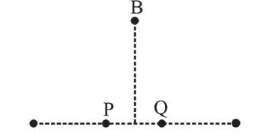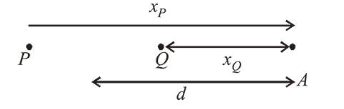# In the figure below, P and Q`
Question:

In the figure below, $\mathrm{P}$ and $\mathrm{Q}$ are two equally intense coherent sources emitting radiation of wavelength $20 \mathrm{~m}$. The separation between $P$ and $Q$ is $5 \mathrm{~m}$ and the phase of $P$ is ahead of that of Q by $90^{\circ} . \mathrm{A}, \mathrm{B}$ and $\mathrm{C}$ are three distinct points of observation, each equidistant from the midpoint of PQ. The intensities of radiation at $\mathrm{A}, \mathrm{B}, \mathrm{C}$ will be in the ratio :1. $0: 1: 4$

2. $2: 1: 0$

3. $0: 1: 2$

4. $4: 1: 0$

Correct Option: , 2

Solution:

(2) For (A)$x_{P}-x_{Q}=(d+2.5)-(d-2.5)=5 \mathrm{~m}$

Phase difference $\Delta \phi$ due to path difference

$=\frac{2 \pi}{\lambda}(\Delta x)=\frac{2 \pi}{20}(5)=\frac{\pi}{2}$

At $A, Q$ is ahead of $P$ by path, as wave emitted by $Q$ reaches before wave emitted by $P$.

$\therefore$ Total phase difference at $A \frac{\pi}{2}-\frac{\pi}{2}=0$

(due to $P$ being ahead of $Q$ by $90^{\circ}$ )

$I_{A}=I_{1}+I_{2}+2 \sqrt{I_{1}} \sqrt{I_{2}} \cos \Delta \phi$

$=I+I+2 \sqrt{I} \sqrt{I} \cos (0)=4 I$

For $C$,

Path difference, $x_{Q}-x_{P}=5 \mathrm{~m}$

Phase difference $\Delta \phi$ due to path difference

$=\frac{2 \pi}{\lambda}(\Delta x)=\frac{2 \pi}{20}(5)=\frac{\pi}{2}$

Total phase difference at $C=\frac{\pi}{2}+\frac{\pi}{2}=\pi$

$I_{\text {net }}=I_{1}+I_{2}+2 \sqrt{I_{1}} \sqrt{I_{2}} \cos (\Delta \phi)$

$=I+I+2 \sqrt{I} \sqrt{I} \cos (\pi)=0$

For $B$,

Path difference, $x_{P}-x_{Q}=0$

Phase difference, $\Delta \phi=\frac{\pi}{2}$

(due to $P$ being ahead of $Q$ by $90^{\circ}$ )

$I_{B}=I+I+2 \sqrt{I} \sqrt{I} \cos \frac{\pi}{2}=2 I$

Therefore intensities of radiation at $A, B$ and $C$ will be in the ratio

$I_{A}: I_{B}: I_{C}=4 I: 2 I: 0=2: 1: 0$